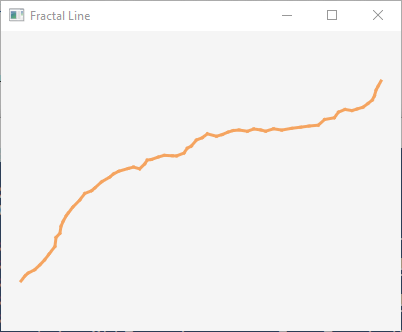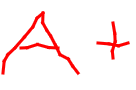created 01/01/03; revised 06/23/2016, JavaFX version: 07/04/2018

# Chapter 95 Programming Exercises

## Exercise 1 — Changing Scale with Recursive Rectangles

Modify the rectangle program by changing how the scale changes each time `fillGroup()` is called. The only requirement is that scale becomes smaller each time. Different types of changes produce different visual effects.

## Exercise 2 — Changing Colors with Recursive Rectangles

Modify the recursive nested rectangle program so that each rectangle is a different color. One way to do this is to pick a random color for the stroke of each rectangle. Another way is to base the color on the current scale, somehow.

Perhaps set the fill color of each rectangle so that solid rectangles are drawn.

See chapter 120 for details on JavaFX class `Color`.

## Exercise 3 — Smallest to Largest Rectangle

Modify the recursive nested rectangle program so that the rectangles are added to the scene graph from smallest to largest (and so are drawn in the same order.)

## Exercise 4 — Twisting Each Recursive Rectangle

Give each rectangle a slight twist by using `setRotate()` to set its rotation property. (See chapter 121.) You may need to increase the spacing between rectangles to prevent overlap if the rotation is large. Base the rotation on the current scale, or use some other means.## Exercise 5 — Fractal LineModify the recursive line drawing program so that the midpoint of each line is dithered a little bit. So the first half of a line extends from a starting point to a dithered midpoint, and the second half extends from that dithered midpoint to the end. And so on down, recursively, until a line is short enough to draw it immediately between each endpoint.

Calculate the midpoint accurately (as before) but then add a random dither amount (negative or positive) to the midpoint X and another random dither amount to the midpoint Y. Base the amount of dither on the current length of the line. If the line length is (say) 100, the dither of the midpoint X might be +8.4 and the dither of the midpoint Y might be -3.3.

Use a `Random` object and `nextDouble()` to calculate the dither. You only need one `Random` object. Construct it in `start()`. It is a common mistake to construct a `Random` object each time a random number is needed, but object construction is expensive in terms of time and machine cycles and you would be constructing many objects that are only used once. Also, since the `Random` objects are constructed very close together in time they may be initialized the same and produce the same number in consecutive calls.

Such lines are fractals. They are sometimes used to give a hand-drawn look to lines. Here is the grade you should expect to see on your paper if you do this assignment correctly: